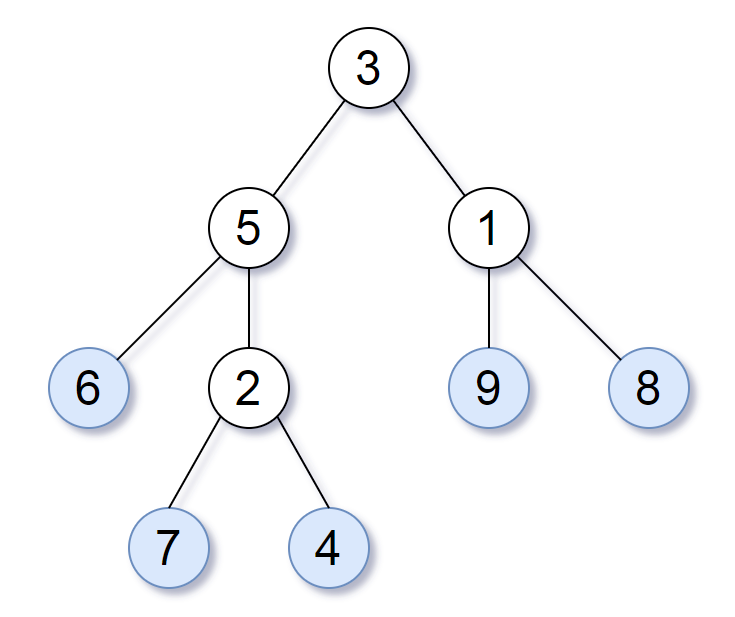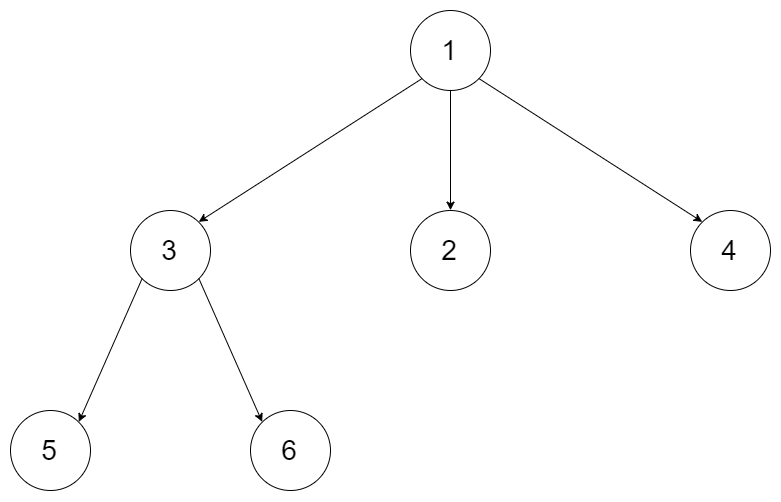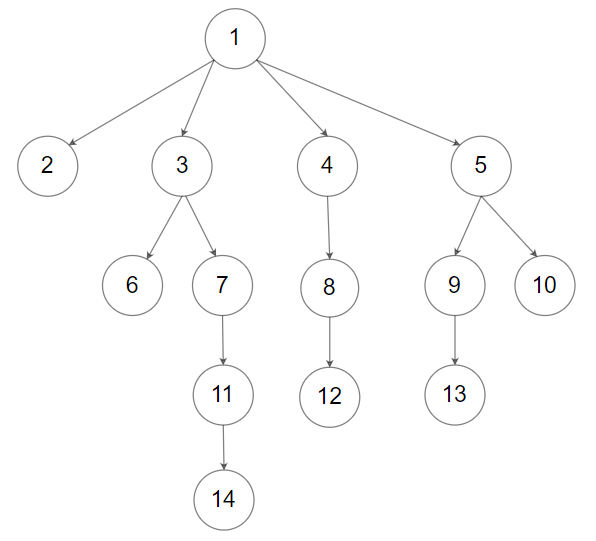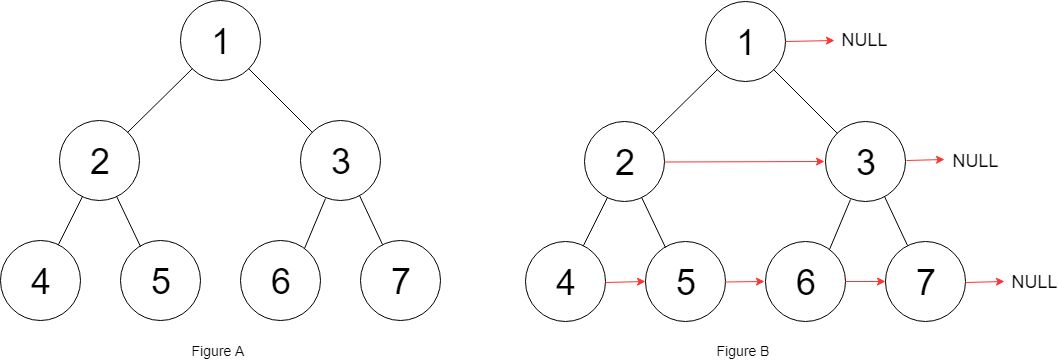# leetcode 332. Reconstruct Itinerary

## 题目描述

Given a list of airline tickets represented by pairs of departure and arrival
airports `[from, to]`, reconstruct the itinerary in order. All of the tickets
belong to a man who departs from `JFK`. Thus, the itinerary must begin with
`JFK`.

Note:

1. If there are multiple valid itineraries, you should return the itinerary that has the smallest lexical order when read as a single string. For example, the itinerary `["JFK", "LGA"]` has a smaller lexical order than `["JFK", "LGB"]`.
2. All airports are represented by three capital letters (IATA code).
3. You may assume all tickets form at least one valid itinerary.

Example 1:

``````Input:[["MUC", "LHR"], ["JFK", "MUC"], ["SFO", "SJC"], ["LHR", "SFO"]]
Output:["JFK", "MUC", "LHR", "SFO", "SJC"]``````

Example 2:

``````Input:[["JFK","SFO"],["JFK","ATL"],["SFO","ATL"],["ATL","JFK"],["ATL","SFO"]]
Output:["JFK","ATL","JFK","SFO","ATL","SFO"]
Explanation: Another possible reconstruction is ["JFK","SFO","ATL","JFK","ATL","SFO"].
But it is larger in lexical order.``````

Tags: Depth-first Search, Graph

Difficulty: Medium



# leetcode 679. 24 Game

## 题目描述

You have 4 cards each containing a number from 1 to 9. You need to judge
whether they could operated through `*`, `/`, `+`, `-`, `(`, `)` to get the
value of 24.

Example 1:

``````Input: [4, 1, 8, 7]
Output: True
Explanation: (8-4) * (7-1) = 24``````

Example 2:

``````Input: [1, 2, 1, 2]
Output: False``````

Note:

1. The division operator `/` represents real division, not integer division. For example, 4 / (1 - 2/3) = 12.
2. Every operation done is between two numbers. In particular, we cannot use `-` as a unary operator. For example, with `[1, 1, 1, 1]` as input, the expression `-1 - 1 - 1 - 1` is not allowed.
3. You cannot concatenate numbers together. For example, if the input is `[1, 2, 1, 2]`, we cannot write this as 12 + 12.

Tags: Depth-first Search

Difficulty: Hard



# leetcode 778. Swim in Rising Water

## 题目描述

On an N x N `grid`, each square `grid[i][j]` represents the elevation at that
point `(i,j)`.

Now rain starts to fall. At time `t`, the depth of the water everywhere is
`t`. You can swim from a square to another 4-directionally adjacent square if
and only if the elevation of both squares individually are at most `t`. You
can swim infinite distance in zero time. Of course, you must stay within the
boundaries of the grid during your swim.

You start at the top left square `(0, 0)`. What is the least time until you
can reach the bottom right square `(N-1, N-1)`?

Example 1:

``````Input: [[0,2],[1,3]]
Output: 3
Explanation:
At time 0, you are in grid location (0, 0).
You cannot go anywhere else because 4-directionally adjacent neighbors have a higher elevation than t = 0.

You cannot reach point (1, 1) until time 3.
When the depth of water is 3, we can swim anywhere inside the grid.``````

Example 2:

``````Input: [[0,1,2,3,4],[24,23,22,21,5],[12,13,14,15,16],[11,17,18,19,20],[10,9,8,7,6]]
Output: 16
Explanation:
**0  1  2  3  4**
24 23 22 21  **5**
**12 13 14 15 16**
**11** 17 18 19 20
**10  9  8  7  6**

The final route is marked in bold.
We need to wait until time 16 so that (0, 0) and (4, 4) are connected.``````

Note:

1. `2 <= N <= 50`.
2. grid[i][j] is a permutation of [0, …, N*N - 1].

Tags: Binary Search, Heap, Depth-first Search, Union Find

Difficulty: Hard



# leetcode 1028. Recover a Tree From Preorder Traversal

## 题目描述

We run a preorder depth first search on the `root` of a binary tree.

At each node in this traversal, we output `D` dashes (where `D` is the depth
of this node), then we output the value of this node. (If the depth of a
node is`D`, the depth of its immediate child is `D+1`. The depth of the root
node is `0`.)

If a node has only one child, that child is guaranteed to be the left child.

Given the output `S` of this traversal, recover the tree and return its
`root`.

Example 1:

![](https://assets.leetcode.com/uploads/2019/04/08/recover-a-tree-from-
preorder-traversal.png)

``````Input: "1-2--3--4-5--6--7"
Output: [1,2,5,3,4,6,7]``````

Example 2:

![](https://assets.leetcode.com/uploads/2019/04/11/screen-
shot-2019-04-10-at-114101-pm.png)

``````Input: "1-2--3---4-5--6---7"
Output: [1,2,5,3,null,6,null,4,null,7]``````

Example 3:

![](https://assets.leetcode.com/uploads/2019/04/11/screen-
shot-2019-04-10-at-114955-pm.png)

``````Input: "1-401--349---90--88"
Output: [1,401,null,349,88,90]``````

Note:

• The number of nodes in the original tree is between `1` and `1000`.
• Each node will have a value between `1` and `10^9`.

Tags: Tree, Depth-first Search

Difficulty: Hard



# leetcode 200. Number of Islands

## 题目描述

Given a 2d grid map of `'1'`s (land) and `'0'`s (water), count the number of
islands. An island is surrounded by water and is formed by connecting adjacent
lands horizontally or vertically. You may assume all four edges of the grid
are all surrounded by water.

Example 1:

``````Input:
11110
11010
11000
00000

Output:  1``````

Example 2:

``````Input:
11000
11000
00100
00011

Output: 3``````

Tags: Depth-first Search, Breadth-first Search, Union Find

Difficulty: Medium



# leetcode 872. Leaf-Similar Trees

## 题目描述

Consider all the leaves of a binary tree. From left to right order, the
values of those leaves form a leaf value sequence.For example, in the given tree above, the leaf value sequence is ```(6, 7, 4, 9, 8)```.

Two binary trees are considered leaf-similar if their leaf value sequence
is the same.

Return `true` if and only if the two given trees with head nodes `root1` and
`root2` are leaf-similar.

Note:

• Both of the given trees will have between `1` and `100` nodes.

Tags: Tree, Depth-first Search

Difficulty: Easy

# leetcode 559. Maximum Depth of N-ary Tree

## 题目描述

Given a n-ary tree, find its maximum depth.

The maximum depth is the number of nodes along the longest path from the root
node down to the farthest leaf node.

Nary-Tree input serialization is represented in their level order traversal,
each group of children is separated by the null value (See examples).

Example 1:Input: root = [1,null,3,2,4,null,5,6] Output: 3

Example 2:Input: root = [1,null,2,3,4,5,null,null,6,7,null,8,null,9,10,null,null,11,null,12,null,13,null,null,14] Output: 5

Constraints:

• The depth of the n-ary tree is less than or equal to `1000`.
• The total number of nodes is between `[0, 10^4]`.

Tags: Tree, Depth-first Search, Breadth-first Search

Difficulty: Easy

# leetcode 897. Increasing Order Search Tree

## 题目描述

Given a binary search tree, rearrange the tree in in-order so that the
leftmost node in the tree is now the root of the tree, and every node has no
left child and only 1 right child.

``````**Example 1:**
Input: [5,3,6,2,4,null,8,1,null,null,null,7,9]

5
/ \
3    6
/ \    \
2   4    8
/        / \
1        7   9

Output: [1,null,2,null,3,null,4,null,5,null,6,null,7,null,8,null,9]

1
\
2
\
3
\
4
\
5
\
6
\
7
\
8
\
9  ``````

Note:

1. The number of nodes in the given tree will be between 1 and 100.
2. Each node will have a unique integer value from 0 to 1000.

Tags: Tree, Depth-first Search

Difficulty: Easy

# leetcode 116. Populating Next Right Pointers in Each Node

## 题目描述

You are given a perfect binary tree where all leaves are on the same
level, and every parent has two children. The binary tree has the following
definition:
struct Node { int val; Node *left; Node *right; Node *next; }

Populate each next pointer to point to its next right node. If there is no
next right node, the next pointer should be set to `NULL`.

Initially, all next pointers are set to `NULL`.

Follow up:

• You may only use constant extra space.
• Recursive approach is fine, you may assume implicit stack space does not count as extra space for this problem.

Example 1:Input: root = [1,2,3,4,5,6,7] Output: [1,#,2,3,#,4,5,6,7,#] Explanation: Given the above perfect binary tree (Figure A), your function should populate each next pointer to point to its next right node, just like in Figure B. The serialized output is in level order as connected by the next pointers, with ‘#’ signifying the end of each level.

Constraints:

• The number of nodes in the given tree is less than `4096`.
• `-1000 <= node.val <= 1000`

Tags: Tree, Depth-first Search

Difficulty: Medium

# leetcode 114. Flatten Binary Tree to Linked List

## 题目描述

Given a binary tree, flatten it to a linked list in-place.

For example, given the following tree:

``````    1
/ \
2   5
/ \   \
3   4   6``````

The flattened tree should look like:

``````1
\
2
\
3
\
4
\
5
\
6``````

Tags: Tree, Depth-first Search

Difficulty: Medium

:D 一言句子获取中...